# Different ways to create Pandas Dataframe

Pandas DataFrame is a 2-dimensional labeled data structure with columns of potentially different types. It is generally the most commonly used pandas object.

Pandas DataFrame can be created in multiple ways. Let’s discuss different ways to create a DataFrame one by one.

Method #1: Creating Pandas DataFrame from lists of lists.

 `# Import pandas library ` `import` `pandas as pd ` ` `  `# initialize list of lists ` `data ``=` `[[``'tom'``, ``10``], [``'nick'``, ``15``], [``'juli'``, ``14``]] ` ` `  `# Create the pandas DataFrame ` `df ``=` `pd.DataFrame(data, columns ``=` `[``'Name'``, ``'Age'``]) ` ` `  `# print dataframe. ` `df `

Output: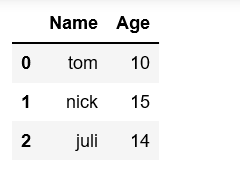Method #2: Creating DataFrame from dict of narray/lists

To create DataFrame from dict of narray/list, all the narray must be of same length. If index is passed then the length index should be equal to the length of arrays. If no index is passed, then by default, index will be range(n) where n is the array length.

 `# Python code demonstrate creating  ` `# DataFrame from dict narray / lists  ` `# By default addresses. ` ` `  `import` `pandas as pd ` ` `  `# intialise data of lists. ` `data ``=` `{``'Name'``:[``'Tom'``, ``'nick'``, ``'krish'``, ``'jack'``], ``'Age'``:[``20``, ``21``, ``19``, ``18``]} ` ` `  `# Create DataFrame ` `df ``=` `pd.DataFrame(data) ` ` `  `# Print the output. ` `df `

Output: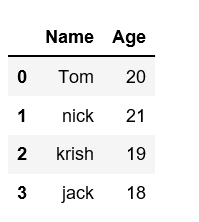Method #3: Creates a indexes DataFrame using arrays.

 `# Python code demonstrate creating  ` `# pandas DataFrame with indexed by  ` ` `  `# DataFrame using arrays. ` `import` `pandas as pd ` ` `  `# initialise data of lists. ` `data ``=` `{``'Name'``:[``'Tom'``, ``'Jack'``, ``'nick'``, ``'juli'``], ``'marks'``:[``99``, ``98``, ``95``, ``90``]} ` ` `  `# Creates pandas DataFrame. ` `df ``=` `pd.DataFrame(data, index ``=``[``'rank1'``, ``'rank2'``, ``'rank3'``, ``'rank4'``]) ` ` `  `# print the data ` `df `

Output:Method #4: Creating Dataframe from list of dicts

Pandas DataFrame can be created by passing lists of dictionaries as a input data. By default dictionary keys taken as columns.

 `# Python code demonstrate how to create  ` `# Pandas DataFrame by lists of dicts. ` `import` `pandas as pd ` ` `  `# Initialise data to lists. ` `data ``=` `[{``'a'``: ``1``, ``'b'``: ``2``, ``'c'``:``3``}, {``'a'``:``10``, ``'b'``: ``20``, ``'c'``: ``30``}] ` ` `  `# Creates DataFrame. ` `df ``=` `pd.DataFrame(data) ` ` `  `# Print the data ` `df `

Output: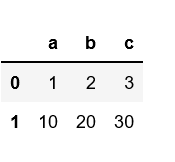Another example to create pandas DataFrame by passing lists of dictionaries and row indexes.

 `# Python code demonstrate to create ` `# Pandas DataFrame by passing lists of  ` `# Dictionaries and row indices. ` `import` `pandas as pd ` ` `  `# Intitialise data of lists  ` `data ``=` `[{``'b'``: ``2``, ``'c'``:``3``}, {``'a'``: ``10``, ``'b'``: ``20``, ``'c'``: ``30``}] ` ` `  `# Creates padas DataFrame by passing  ` `# Lists of dictionaries and row index. ` `df ``=` `pd.DataFrame(data, index ``=``[``'first'``, ``'second'``]) ` ` `  `# Print the data ` `df `

Output: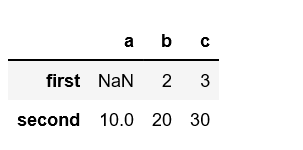Another example to create pandas DataFrame from lists of dictionaries with both row index as well as column index.

 `# Python code demonstrate to create a ` `# Pandas DataFrame with lists of  ` `# dictionaries as well as  ` `# row and column indexes. ` `  `  `import` `pandas as pd ` `  `  `# Intitialise lists data. ` `data ``=` `[{``'a'``: ``1``, ``'b'``: ``2``}, {``'a'``: ``5``, ``'b'``: ``10``, ``'c'``: ``20``}] ` `  `  `# With two column indices, values same  ` `# as dictionary keys ` `df1 ``=` `pd.DataFrame(data, index ``=``[``'first'``, ``'second'``], columns ``=``[``'a'``, ``'b'``]) ` `  `  `# With two column indices with  ` `# one index with other name ` `df2 ``=` `pd.DataFrame(data, index ``=``[``'first'``, ``'second'``], columns ``=``[``'a'``, ``'b1'``]) ` `  `  `# print for first data frame ` `print` `(df1, ``"\n"``) ` `  `  `# Print for second DataFrame. ` `print` `(df2) `

Output: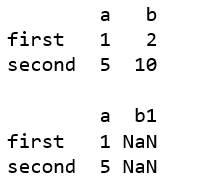Method #5: Creating DataFrame using `zip()` function.

Two lists can be merged by using `list(zip())` function. Now, create the pandas DataFrame by calling `pd.DataFrame() `function.

 `# Python program to demonstrate creating  ` `# pandas Datadaframe from lists using zip.  ` `   `  `import` `pandas as pd  ` `   `  `# List1  ` `Name ``=` `[``'tom'``, ``'krish'``, ``'nick'``, ``'juli'``]  ` `   `  `# List2  ` `Age ``=` `[``25``, ``30``, ``26``, ``22``]  ` `   `  `# get the list of tuples from two lists.  ` `# and merge them by using zip().  ` `list_of_tuples ``=` `list``(``zip``(Name, Age))  ` `   `  `# Assign data to tuples.  ` `list_of_tuples   ` ` `  ` `  `# Converting lists of tuples into  ` `# pandas Dataframe.  ` `df ``=` `pd.DataFrame(list_of_tuples, columns ``=` `[``'Name'``, ``'Age'``])  ` `    `  `# Print data.  ` `df  `

Output: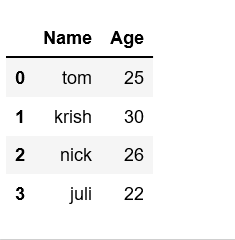Method #6: Creating DataFrame from Dicts of series.

To create DataFrame from Dicts of series, dictionary can be passed to form a DataFrame. The resultant index is the union of all the series of passed indexed.

 `# Python code demonstrate creating ` `# Pandas Dataframe from Dicts of series. ` ` `  `import` `pandas as pd ` ` `  `# Intialise data to Dicts of series. ` `d ``=` `{``'one'` `: pd.Series([``10``, ``20``, ``30``, ``40``], index ``=``[``'a'``, ``'b'``, ``'c'``, ``'d'``]), ` `      ``'two'` `: pd.Series([``10``, ``20``, ``30``, ``40``], index ``=``[``'a'``, ``'b'``, ``'c'``, ``'d'``])} ` ` `  `# creates Dataframe. ` `df ``=` `pd.DataFrame(d) ` ` `  `# print the data. ` `df `

Output: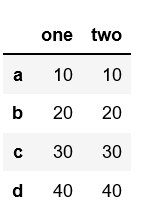My Personal Notes arrow_drop_upCheck out this Author's contributed articles.

If you like GeeksforGeeks and would like to contribute, you can also write an article using contribute.geeksforgeeks.org or mail your article to contribute@geeksforgeeks.org. See your article appearing on the GeeksforGeeks main page and help other Geeks.

Please Improve this article if you find anything incorrect by clicking on the "Improve Article" button below.

Article Tags :

8

Please write to us at contribute@geeksforgeeks.org to report any issue with the above content.